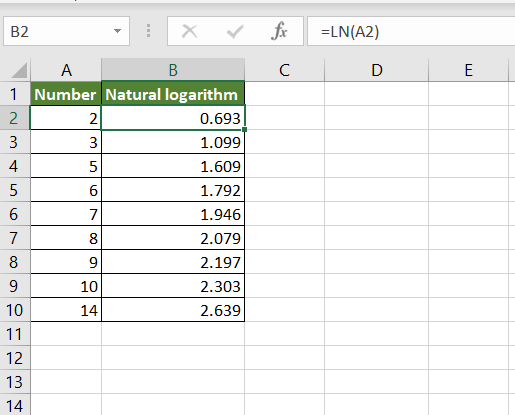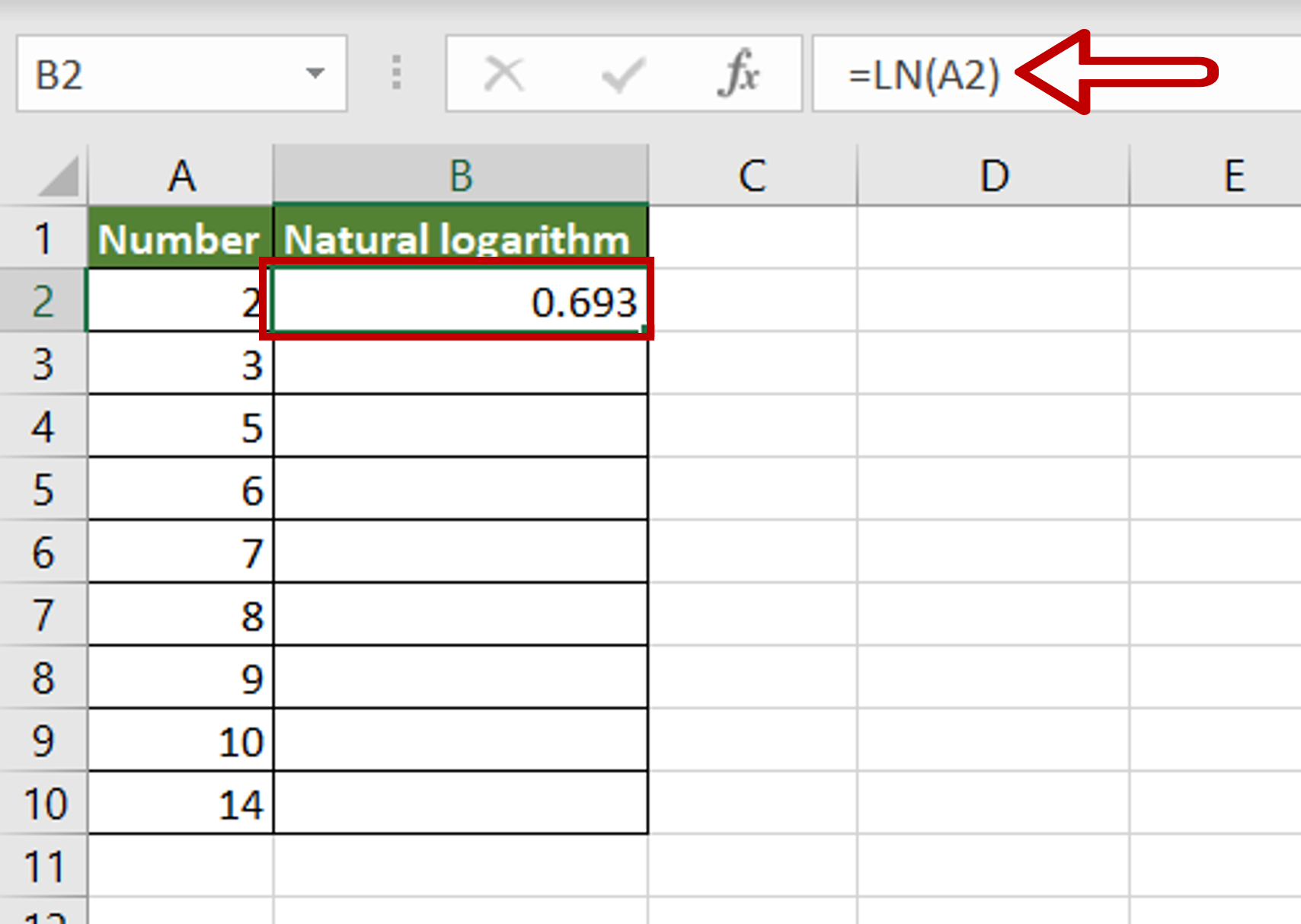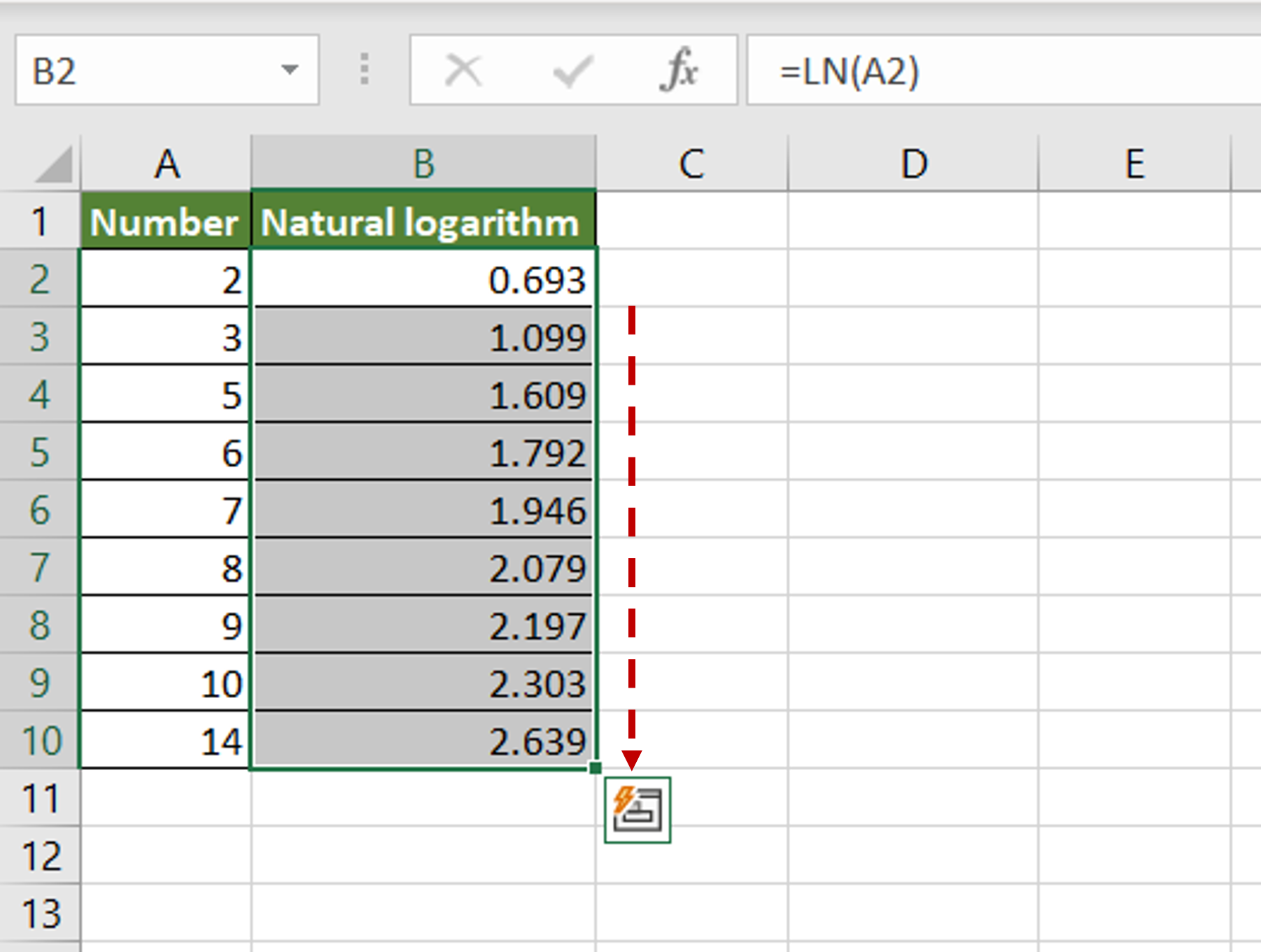# How to take the natural log of a column in Excel

You can watch a video tutorial here.Excel has several operators and functions that can be used to perform basic mathematical operations. One such operation is finding the natural log of a number. A natural logarithm is the logarithm of a number to the base e (Euler’s number). In Excel, this can be done using the LN() function.

1. LN() function: this returns the natural logarithm of a number
a. Syntax: LN(number)
i. number: the number of which the natural logarithm is to be computed

### Step 1 – Create the formula– Select the destination cell
– Type the formula using cell references:
=LN(number)
– Press Enter

### Step 2 – Copy the formula– Using the fill handle from the first cell, drag the formula to the remaining cells
OR
a. Select the cell with the formula and press Ctrl+C or choose Copy from the context menu (right-click)
b. Select the rest of the cells in the column and press Ctrl+V or choose Paste from the context menu (right-click)

### Step 3 – Check the result– The natural logarithm of each number in the column is displayed